# Nuclear Cross section

When projectile particles bombard with the target nucleus, these are different possible ways of removing of particles from incident beam. The particles ray be scattered or may be absorbed by the target nucleus. The chance of occurrence of a particular type of nuclear interaction is represented in terms of area. The effective area offered by the target nucleus for an incident particle for a particular type of nuclear interaction is known as nuclear cross section. So, the particle bombarding to this area interacts the nucleus. Higher the cross section, more likely is the nuclear reaction to occur.

The nuclear cross section is defined as the probability that an event (scattering, absorption) may occur when a single target is exposed to the beam of incident particles having flux (number of particles per unit area) . or The probability that an event may occur when a incident particles is shot perpendicularly to the target containing single nucleus per unit area.

The total nuclear cross section is the sum of partial cross sections like scattering cross section and reaction cross section.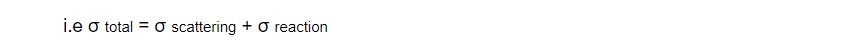The scattering of particle by the target may be either by inelastic or by elastic scattering. So, the scattering cross section can be divided further two partial cross sections.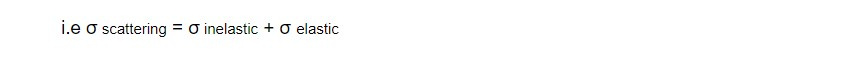Similarly Nuclear reaction cross section can also be divided into partial cross section representing the probability of different possible nuclear reactions.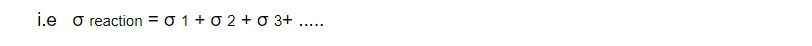Determination of Nuclear cross reaction: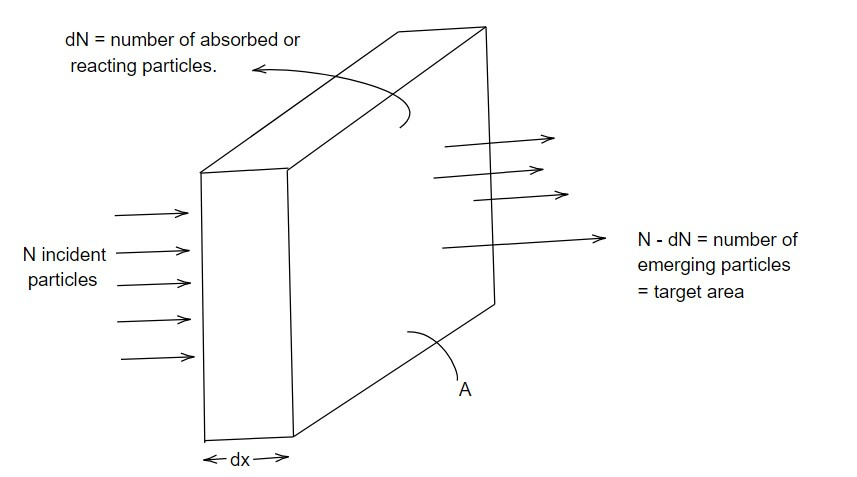Let us consider a slab of surface area A and the thickness dx of a particular target material.

Then, total volume of the slab = Adx

If n is number of target nuclei per unit volume then total number of target nuclei in the slab = nAdx

If σ is the nuclear cross section offered by each nucleus to the incident particle for the nuclear reaction then

Total cross section offered by the slab = σ(nAdx)

Consider N be the number of incident particles survived after passing x thickness of target material and dN be the number of particles absorbed or reacting within the small thickness dx, thenSo σ (microscopic cross section) is fraction of particles absorbed or reacting per target nucleus in unit area. It is measured in the unit of area barn where

Since dN is number of particles interacting with target nuclei within thickness dx, it can also be considered as number of incident particles removed from incident beam.

So equation 1 can be written as

- dN/ N = σndx ..........2

negative sign indicates that the number of bombarding particles goes on decreasing.

Integrating above equation 2,loge N - log e No = σnx

or, log e (N / No) = -σ nx

The number of particles remained without interaction after passing thickness 'x' decreases exponentially with thickness.

The quantity Σ = σn is called as macroscopic cross section offered by all nuclei within unit volume.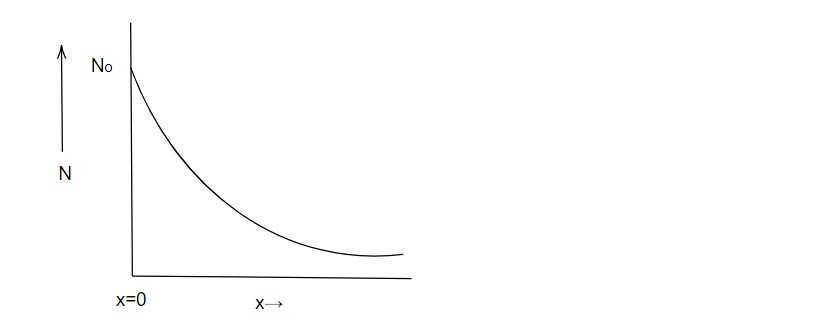Mean free path and macroscopic cross section:

Mean free path of the incident particles is defines as the average distance travelled by them before interacting with target nuclei. It is denoted by λ.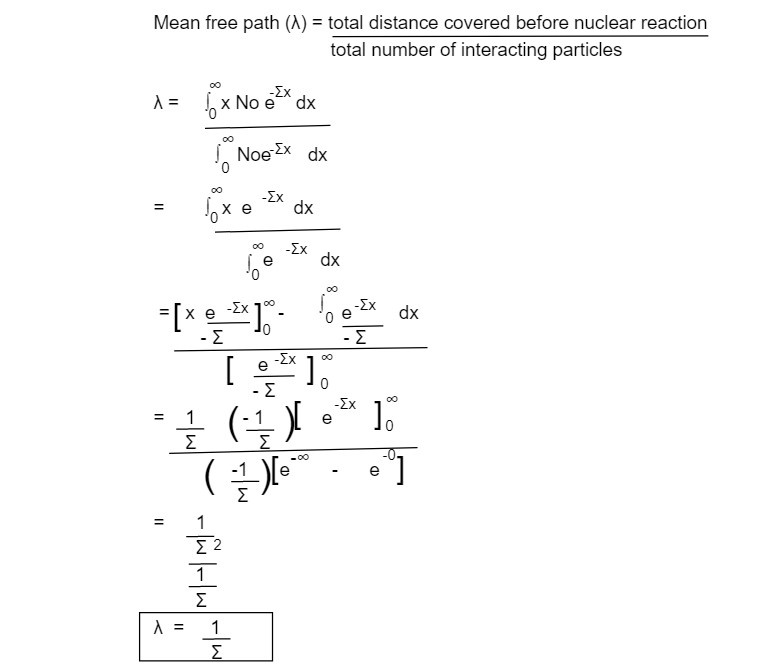The mean free path is the reciprocal of macroscopic cross section.

Discovery of Neutron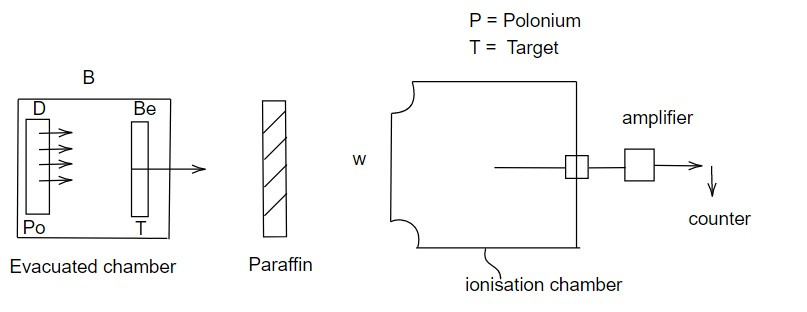In 1930, Bethe and Becker bombarded α particles on the target of Berylium and found highly penetrating chargeless particles supposed to be hard gamma ray photon produced according to the reaction.

The energy of gamma ray photon was found to be 7 Mev which has the high energy than that known at that time. Curie and Juliot allowed the radiations emitted from above process to incident on the hydrogenous compounds like paraffin. The protons were found to be produced having energy 6 Mev. It was considered that the proton were ejected due to the collision between photon and proton. But to eject proton of 6 Mev energy by proton, it must have at least 60 Mev energy which didn't matter 7 MeV of energy of radiation.

To avoid all these discrepancies Chadwick in 1932 proposed that a chargeless particle having mass nearly equal to that of proton must be liberated according to the reaction.

So that neutron of 7 MeV energy can knock out proton of about same mass and energy.

Chadwick later performed an experiment to demonstrate the existence of neutron.

In his experiment, the α particle emitted from polonium as a radioactive source were allowed to incident on the target of Beryllium.

The whole apparatus were kept within a evacuated chamber B. The particle emitted from the reaction were then allowed to enter into the ionisation chamber (C) through window (W). The ionisation chamber was connected with amplifier and then to counter to count the ionising particles.

Since neutron are chargeless, only few counts registered were supposed to be due to ions produced by collision of neutron on the thin wall of chamber. When a load sheet of 2 cm thickness was placed before window (w), there was no charge in the counter rate. So, neutron were highly penetrating. But when paraffin was kept, the counts rate was found to be increased which is due to the protons from paraffin due to neutron bombardment.

This note is a part of the Physics Repository.